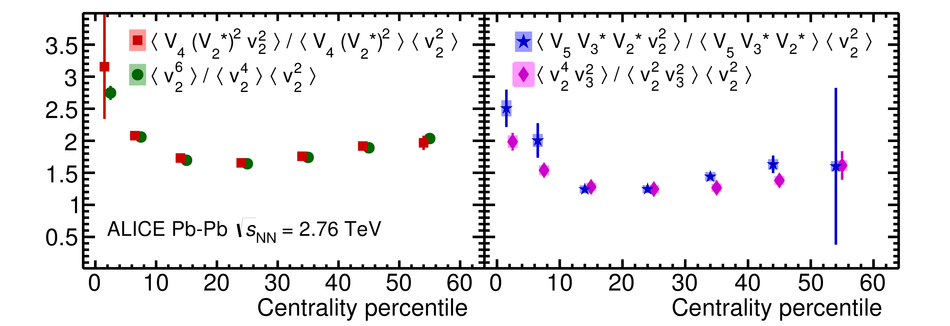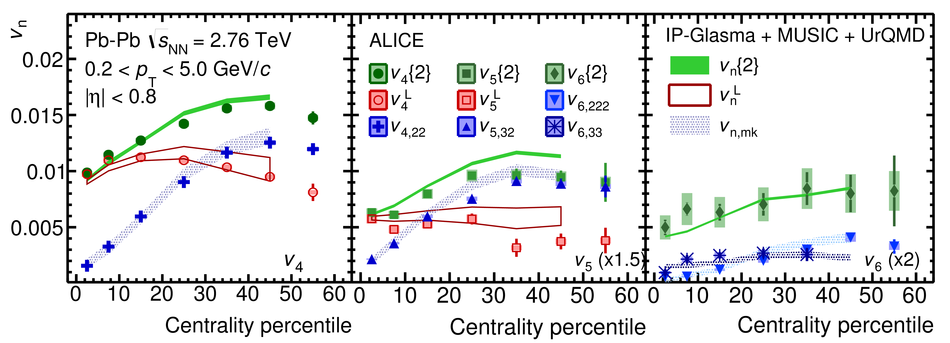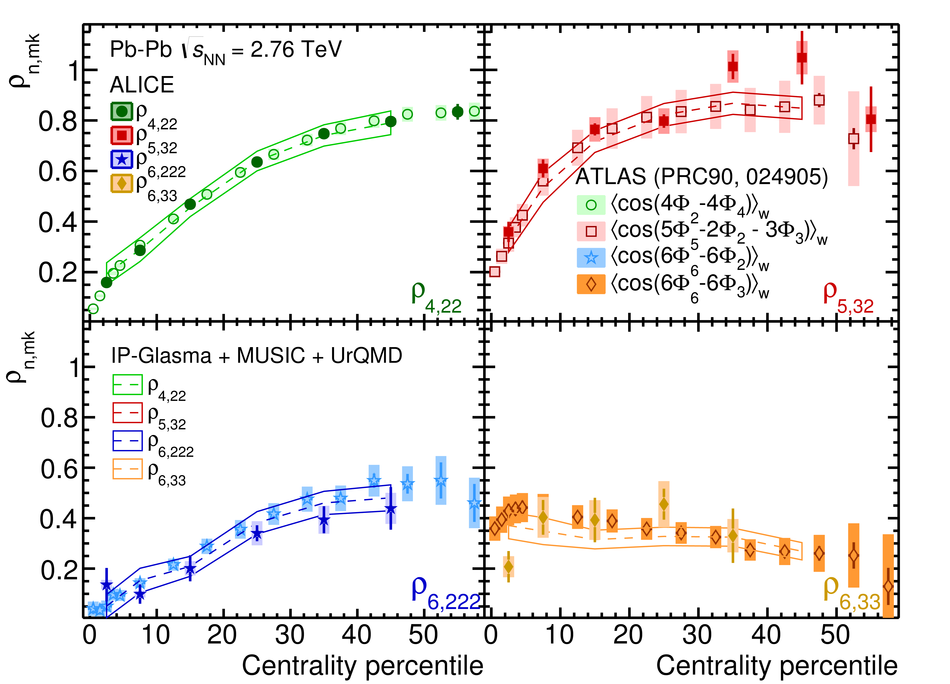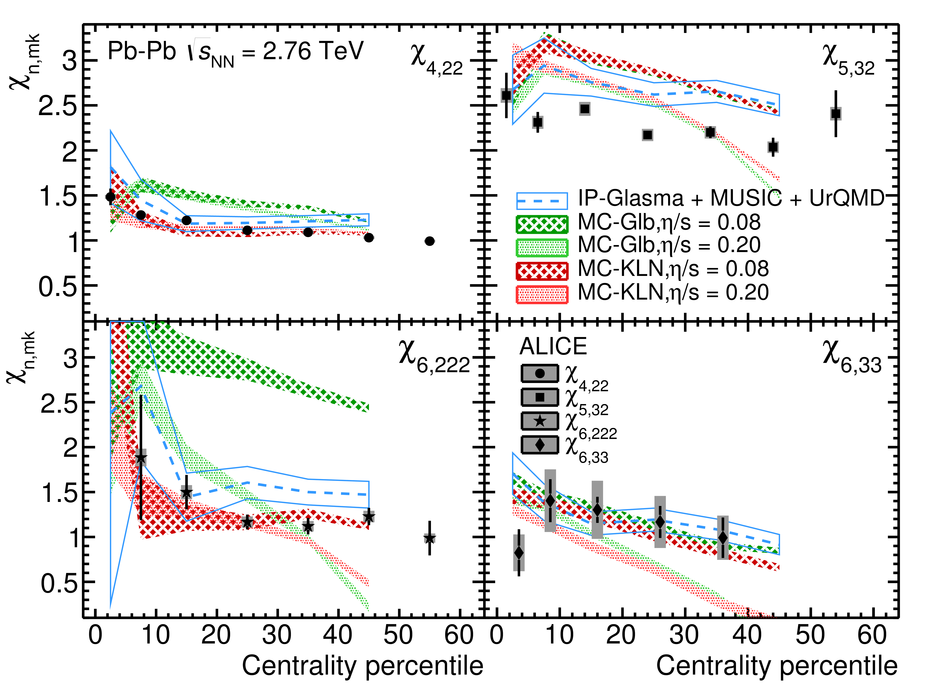# Linear and non-linear flow modes in Pb-Pb collisions at $\sqrt{s_{\rm NN}} =$ 2.76 TeV

The second and the third order anisotropic flow, $V_{2}$ and $V_3$, are mostly determined by the corresponding initial spatial anisotropy coefficients, $\varepsilon_{2}$ and $\varepsilon_{3}$, in the initial density distribution. In addition to their dependence on the same order initial anisotropy coefficient, higher order anisotropic flow, $V_n$ ($n > 3$), can also have a significant contribution from lower order initial anisotropy coefficients, which leads to mode-coupling effects. In this Letter we investigate the linear and non-linear modes in higher order anisotropic flow $V_n$ for $n=4$, 5, and 6 in Pb-Pb collisions at $\sqrt{s_{\rm NN}}=2.76$ TeV with the ALICE detector at the Large Hadron Collider. The measurements are done for particles in the pseudorapidity range $|\eta| <~ 0.8$ and the transverse momentum range $0.2 <~ p_{\rm T} <~ 5.0$ GeV/$c$ as a function of collision centrality. The results are compared with theoretical calculations and provide important constraints on the initial conditions, including initial spatial geometry and its fluctuations, as well as the ratio of the shear viscosity to entropy density of the produced system.

Figures

## Figure 1

 Study of relationship between linear and non-linear modes in higher order anisotropic flow in Pb--Pb collisions at $\sqrt{s_{_{\rm NN}}} = 2.76$ TeV, according to Eqs.~(\ref{eq:equality1}) and (\ref{eq:equality2}).## Figure 2

 Centrality dependence of $v_4$ (left), $v_5$ (middle) and $v_6$ (right) in Pb--Pb collisions at $\sqrt{s_{_{\rm NN}}} = 2.76$ TeV. Contributions from linear and non-linear modes are presented with open and solid markers, respectively. The hydrodynamic calculations from ${\tt IP}$-${\tt Glasma+MUSIC+UrQMD}$~ are shown for comparison.## Figure 3

 Centrality dependence of $\rho_{n,mk}$ in Pb--Pb collisions at $\sqrt{s_{_{\rm NN}}} = 2.76$ TeV. ATLAS measurements based on the event-plane correlation~ are presented with open markers. The hydrodynamic calculations from ${\tt IP}$-${\tt Glasma+MUSIC+UrQMD}$~ are shown with open bands.## Figure 4

 Centrality dependence of $\chi$ in Pb--Pb collisions at $\sqrt{s_{_{\rm NN}}} = 2.76$ TeV. Hydrodynamic calculations from ${\tt VISH2+1}$~ are shown in shaded areas and the one from ${\tt IP}$-${\tt Glasma+MUSIC+UrQMD}$~ are shown with open bands.